# School year

At the beginning of the school year, 396 notebooks and 252 textbooks are ready to be distributed in the classroom. All pupils receive the same number of notebooks and the same amount of textbooks. How many pupils are there in the class if you know that there are more than 30 and less than 50?

n =  36

### Step-by-step explanation: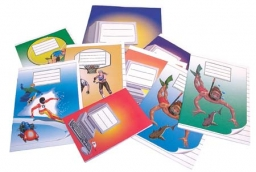Did you find an error or inaccuracy? Feel free to write us. Thank you!Tips to related online calculators
Do you want to calculate least common multiple two or more numbers?
Do you want to perform natural numbers division - find the quotient and remainder?

## Related math problems and questions:

• School booksAt the beginning of the school year, the teacher distributed 480 workbooks and 220 textbooks. How many pupils could have the most in the classroom?
• On Children'sOn Children's Day, the organizers bought 252 chewing gums, 396 candies and 108 lollipops. They want to make as many of the same packages as possible. Advise them what to put in each package and how many packages they can make this way.
• Dining tables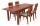In the dining room are tables with 4 chairs, 6 chairs, 8 chairs. How many diners must be at least to occupy all tables (chairs) and diners are more than 50?
• Find unknown numberWhat is the number between 50 and 55 that is divisible by 2,3,6,9?
• TissuesThe store got three kinds of tissues - 132 children, 156 women and 204 men. Tissues each species were packed into boxes after the number of pieces the same for all three types (and greatest). Determine the number, if you know that every box has more than
• LCMWhat is the least common multiple of 5, 50, 14?
• Cash back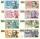After a trip 24 students have left more than 650 but less than 690 CZK (Kc). How much each student receives return when the amount is divisible by the number of pupils?
• AthletesAthletes at the stadium could enter two-steps, three-steps, four-steps, five-steps, six-steps. There were more than 100 but less than 200. How many athletes were there?
• RemaindersIt is given a set of numbers { 170; 244; 299; 333; 351; 391; 423; 644 }. Divide this numbers by number 66 and determine set of remainders. As result write sum of this remainders.
• Trees in alleyThere are four trees in the alley between which the distances are 35m, 15m and 95m. Trees must be laid in the spaces so that the distance is the same and the maximum. How many trees will they put in and what will be the distance between them?
• Lesson exercising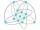The lesson of physical education, pupils are first divided into three groups so that each has the same number. The they redistributed, but into six groups. And again, it was the same number of children in each group. Finally they divided into nine equal g
• LCM of two numberFind the smallest multiple of 63 and 147
• There 14There are 250 people in a museum. 2/5 of the 250 people are girls 3/10 of the 250 people are boys The rest of the 250 people are adults Work out the number of adults in the museum.
• Lamps on playground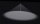The playground has the shape of a rectangle 36 x 50m. After how many meters will he place the lamps on his lighting, if the distances between them are to be the same on both sides if the builders want to use the smallest possible number of lamps?
• Dinosaurs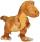More than 30 and less than 60 dinosaurs have met at the pond. A quarter of them bathed and 1/7 saws, and the rest gripped. How many were at the pond? How many were there?On the meadow grazing horses, cows, and sheep, together with less than 200. If cows were 45 times more, horses 60 times more, and sheep 35 times more than there are now, their numbers would equally. How many horses, cows, and sheep are on the meadow togetThere are given numbers A = 135, B = 315. Find the smallest natural number R greater than 1 so that the proportions R:A, R:B are with the remainder 1.